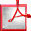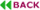Analysis of Short-Circuit Conditions in Logic Circuits

Joã Afonso1 and José Monteiro2
1INESC-ID, Portugal.
jpa@algos.inesc-id.pt
2INESC-ID / IST, U Lisboa, Portugal.
jcm@inesc-id.pt

ABSTRACT

The motivation for this paper is the analysis of input conditions that cause a short-circuit in a logic circuit, that is, that create a direct path from the power supply to ground. We model the logic circuit as a graph where edges represent transistors which are either open or closed, function of the input conditions. From this graph we derive a Quantified Boolean Formula (QBF) problem whose solution identifies the existence of a valid input combination that creates a path in the graph between the pair of nodes that represent the power source and ground, without ever enumerating all input combinations. We build the QBF problem incrementally, minimizing the number of active nodes and hence of possible states. In the end, we obtain a relatively simple CNF expression, function only of the circuit inputs, that is handled by a generic SAT solver. We present results that demonstrate the practical applicability of our method on circuit instances that are intractable by alternative methods.Full Text (PDF)